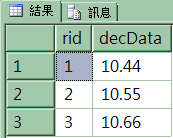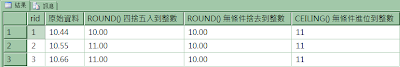## 2010-07-01

### 使用 ROUND、CEILING、FLOOR 函數，執行四捨五入到整數、無條件捨去到整數、無條件進位到整數、無條件捨去到指定的有效位數

```-- 01 建立範例資料表
USE tempdb
GO
CREATE TABLE math01
(rid int IDENTITY(1,1), decData decimal(5,2) )
GO
INSERT math01 VALUES(10.44)
INSERT math01 VALUES(10.55)
INSERT math01 VALUES(10.66)
GO

-- 02 查詢資料表
SELECT * FROM math01
``````--03 使用 ROUND、CEILING 函數，執行四捨五入到整數、無條件捨去到整數、無條件進位到整數
SELECT rid, decData N'原始資料',
ROUND(decData,0) N'ROUND() 四捨五入到整數',
ROUND(decData,0,1) N'ROUND() 無條件捨去到整數',
CEILING(decData) N'CEILING() 無條件進位到整數'
FROM math01
``````--04 使用 ROUND、FLOOR 函數，執行無條件捨去到整數、無條件捨去到指定的有效位數
SELECT rid, decData N'原始資料',
FLOOR(decData) N'FLOOR() 無條件捨去到整數',
ROUND(decData,0,1) N'ROUND() 無條件捨去到整數',
ROUND(decData,1,1) N'ROUND() 無條件捨去到小數點第一位數'
FROM math01
```

CEILING (Transact-SQL)

ROUND (Transact-SQL)

ROUND ( numeric_expression , length [ ,function ] )
ROUND 一律傳回值。如果 length 是負的，且大於小數點前面的位數，ROUND 會傳回 0。

numeric_expression

length

length 必須是 tinyint、smallint 或 int 類型的運算式。

function

FLOOR (Transact-SQL)

CEILING 函數會傳回大於或等於指定的數值運算式之最小整數。

FLOOR 函數會傳回小於或等於指定的數值運算式之最大整數。

CEILING (Transact-SQL)
http://msdn.microsoft.com/zh-tw/library/ms189818.aspx

ROUND (Transact-SQL)
http://technet.microsoft.com/zh-tw/library/ms175003.aspx

FLOOR (Transact-SQL)
http://technet.microsoft.com/zh-tw/library/ms178531.aspx

CEILING 與 FLOOR 的比較
http://msdn.microsoft.com/zh-tw/library/ms190927.aspx

http://sharedderrick.blogspot.com/2009/06/round-sql-server-2008.html